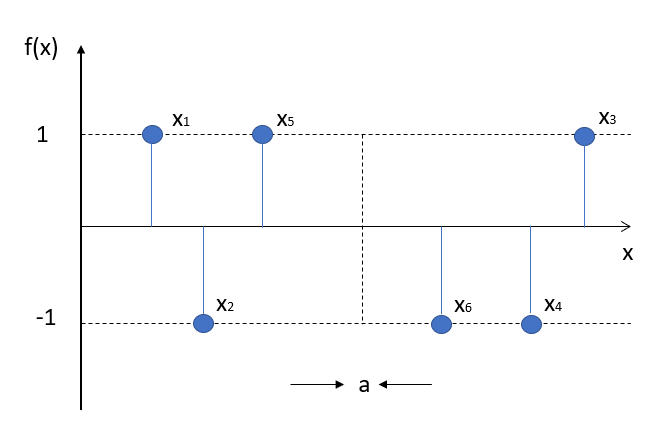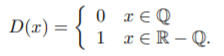# Nowhere Continuous Function

A nowhere continuous function (also called an everywhere discontinuous function) is, perhaps unsurprisingly, not continuous anywhere. Several different types of these functions exist, but they are share in common an alternating, jumping pattern as you move along the x-axis. For example, one point might have a function value of -1, the next point +1, then -1, then +1…and so on.

Although the nowhere continuous function might seem a little odd, the very not continuous function is even stranger: it’s a nowhere continuous function where the absolute values are everywhere continuous.

## Examples of Nowhere Continuous Functions

A simple example (Pahl & Damrath, 2012) is a function with a value of 1 if x is a rational number (ℚ) and -1 is every other real number (ℝ – ℚ). A rational number can be written as a simple fraction (like 3/1 or 2/3) while the irrationals cannot. This means that as you move across the number line, the function inputs jump from irrational to rational and back again. This means that the function outputs form basic sequences <1, -1, 1, -1,…>.Dirichlet’s nowhere continuous function is a well-known nowhere continuous function, defined on ℝ (where ℝ is the set of real numbers): it equals 1 if x is a rational number (denoted by ℚ) and 0 is x isn’t a rational number. In notation (Chen & Smith), that’s:The graph of Dirichlet’s nowhere continuous function is similar to the graph of the simple example above:## References

Chen, J. & Smith, S. Cardinality of the set of real functions with a given continuity set. Retrieved January 10, 2020 from: http://people.sju.edu/~smith/Current_Courses/continuitysetsrev.pdf
Pahl, P. & Damrath, R. (2012). Mathematical Foundations of Computational Engineering: A Handbook. Springer Science & Engineering.

CITE THIS AS:
Stephanie Glen. "Nowhere Continuous Function" From StatisticsHowTo.com: Elementary Statistics for the rest of us! https://www.statisticshowto.com/nowhere-continuous-function/